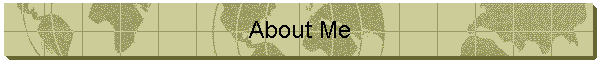This site was last updated 08/09/22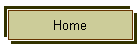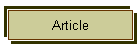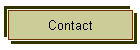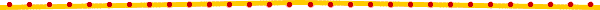Discoverer of prime numbers formula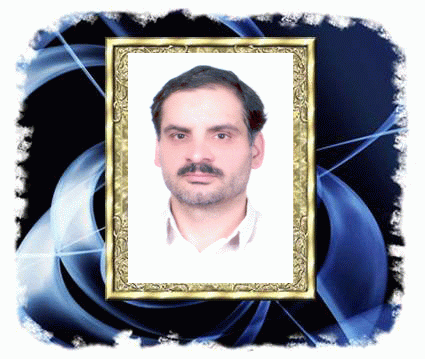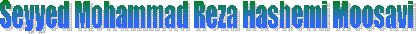Discoverer of prime numbers formula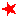Short introduction :This is the Prof. S.M.R Hashemi Moosavi's picture. I have discovered the formula of the prime numbers after 20 years of research finally for the first time in the human history in the year of 2003 and also solved the unsolvable problems related to them.Discovery results :

•    The distinction of the prime numbers.

•    The generating formula of the prime numbers.

•        The definition of the prime numbers set by using the generating function of prime numbers.

•    The generating formula of the Mersenne prime numbers.

•         The determining of the k-th prime number.

•        Solving Riemann zeta equation by using the determining of the number of the prime numbers less than or equal arbitrary number (N) exactly.

•     Study of the guesses of Gold Buch and Hardy.

•     The proof of being infinity of the prime twin couples.Note: the results of this great discovery "the formula of generating prime numbers " has been sent to the most of research centers and universities of the world.Introduction :

I am Seyyed Mohammad Reza Hashemi Moosavi and university professor that I chosen as a superior investigator in superiors and initiators festival in 1383. My academic course is telecommunication electric engineering and I received specialized doctorate of Education (PhD) from Boston university of America and doctorate of mathematics (PhD) from Spain.

researching works :

I have started my researches in mathematics field when I was fourteen. My first research was a flash that one of my mathematics teachers in guidance school caused it. He pronounced a method of mental multiplication of numbers. Impetus of mental multiplication occupied my mind to research for several years. Till in first year of high school, I could obtain a mental \multiplication method for M figures and N figures and it was my first success in research works. My second research which lasted around two years was obtain the method of algebraic and geometric solution in cubic equation that I obtain in fourth year in high school and published in 22nd copy of mathematics teaching development magazine from research and lessons programmer organization. After it I researched seriously. For example calculation of K-th strength for n prime number that I express Sk a determinant which it doesn't need to Bernoulli coefficients or analysis methods. This point published in 16th copy of mathematics teaching development magazine too and then in 1994 it published in spectrum (the university Sheffield) in England. I obtain the calculation of ellipse circumference which has many usages in calculation of integral function and ellipsoid integral in analysis in a perfectly analytic method and it will publish in spectrum magazine. I performed another research like congruence equation solution in table method that it gives answer of every congruence equation with optional coefficient in the shortest time which is possible. My other research was presented a new method with a highest race of calculation for N*N determinant and it published in spectrum magazine in 2003.It is necessary to mention this point that all of these research became pedestal for my next research like obtaining the prime number formula. My other researches are integral expansion to series and calculation of integrals which have N-th power and express in a returning method. These articles published in ''Acquaintance with mathematics '' too and also my other researches that published in different copy of this magazine. My another important and basic researches is solution of fluid equation in N-th degree that has a great usage in engineering sciences and researching center .of course I performed a lot of researches in algebra, analysis , number theory field and other mathematics branches.Home | About Me | Article | Books | Photo Gallery | Contact

This site was last updated 2022-08-09